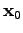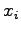# 4. Algebraic Systems

In Chapter 3 the most commonly used discretization schemes were presented using the topological formulation derived in Chapter 2. Further steps have to be accomplished in order to obtain an equation system and consequently an appropriate solution.

Firstly, it has to be remarked that the given formulation of the discretized differential equations only concerns the residua of the respective discretized operations and not the dependences of the unknown variables as required for linear equation systems. For this reason a data structure which is compatible with the specification methods of Section 2.5 is introduced, which stores values as well as the linearized dependences for given variables. A method is shown which automatically derives the linear dependences on the unknown variables and assembles the linearized equations line-wise.

One commonly used method which is especially employed for finite elements, namely the assembly via local shape matrices, is discussed and compared to the line-wise assembly method. Furthermore, difficulties and advantages of these methods are compared to line-wise assembly with a focus on boundaries and interface conditions.

In most cases methods for the solution of nonlinear equation systems rely on linear or linearized equation systems. These systems result from the nonlinear system by a linearization (first order multi-variable Taylor approximation) around a given vectorof linearization. Thus, for each variablea value for the linearization is defined. In the neighborhood of the linearization vector, the respective function can be approximated via the linear (affine) functionusing an appropriately chosen initial vector.

In order to obtain a solution, the resulting equation system has to be solved. This means that the equations have to be assembled into a system matrix and/or a right-hand-side vector.

Subsections
Michael 2008-01-16